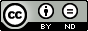The search result changed since you submitted your search request. Documents might be displayed in a different sort order.
• search hit 4 of 808
Back to Result List

## Riemannian Optimization for Advanced Statistical Models with Clustered Data

• Modern decision making in the digital age is highly driven by the massive amount of data collected from different technologies and thus affects both individuals as well as economic businesses. The benefit of using these data and turning them into knowledge requires appropriate statistical models that describe the underlying observations well. Imposing a certain parametric statistical model goes along with the need of finding optimal parameters such that the model describes the data best. This often results in challenging mathematical optimization problems with respect to the model’s parameters which potentially involve covariance matrices. Positive definiteness of covariance matrices is required for many advanced statistical models and these constraints must be imposed for standard Euclidean nonlinear optimization methods which often results in a high computational effort. As Riemannian optimization techniques proved efficient to handle difficult matrix-valued geometric constraints, we consider optimization over the manifold of positive definite matrices to estimate parameters of statistical models. The statistical models treated in this thesis assume that the underlying data sets used for parameter fitting have a clustering structure which results in complex optimization problems. This motivates to use the intrinsic geometric structure of the parameter space. In this thesis, we analyze the appropriateness of Riemannian optimization over the manifold of positive definite matrices on two advanced statistical models. We establish important problem- specific Riemannian characteristics of the two problems and demonstrate the importance of exploiting the Riemannian geometry of covariance matrices based on numerical studies.

Author: Lena Caroline Sembach urn:nbn:de:hbz:385-1-19744 https://doi.org/10.25353/ubtr-xxxx-d39a-3290 Doctoral Thesis English 2023/02/11 Universität Trier Universität Trier, Fachbereich 4 2022/09/21 2023/02/16 Cluster Datenanalyse; Optimierung; Riemannsche Geometrie; Statistisches Modell VII, 169 Blätter VI 169CC BY-ND: Creative-Commons-Lizenz 4.0 International